# US20030097388A1 - Cordic method and architecture applied in vector rotation - Google Patents

Cordic method and architecture applied in vector rotation Download PDF

## Info

Publication number
US20030097388A1
US20030097388A1 US10/138,652 US13865202A US2003097388A1 US 20030097388 A1 US20030097388 A1 US 20030097388A1 US 13865202 A US13865202 A US 13865202A US 2003097388 A1 US2003097388 A1 US 2003097388A1
Authority
US
United States
Prior art keywords
φ
step
respectively
θ
connected
Prior art date
Legal status (The legal status is an assumption and is not a legal conclusion. Google has not performed a legal analysis and makes no representation as to the accuracy of the status listed.)
Granted
Application number
US10/138,652
Other versions
US7047269B2 (en
Inventor
Cheng-Shing Wu
Chia-Ho Pan
An-Yeu Wu
Current Assignee (The listed assignees may be inaccurate. Google has not performed a legal analysis and makes no representation or warranty as to the accuracy of the list.)
Industrial Technology Research Institute
Original Assignee
Industrial Technology Research Institute
Priority date (The priority date is an assumption and is not a legal conclusion. Google has not performed a legal analysis and makes no representation as to the accuracy of the date listed.)
Filing date
Publication date
Priority to TW90127085 priority Critical
Priority to TW90127085A priority patent/TW583579B/en
Application filed by Industrial Technology Research Institute filed Critical Industrial Technology Research Institute
Assigned to INDUSTRIAL TECHNOLOGY RESEARCH INSTITUTE reassignment INDUSTRIAL TECHNOLOGY RESEARCH INSTITUTE ASSIGNMENT OF ASSIGNORS INTEREST (SEE DOCUMENT FOR DETAILS). Assignors: PAN, CHIA-HO, WU, AN-YEU, WU, CHENG-SHING
Publication of US20030097388A1 publication Critical patent/US20030097388A1/en
Application granted granted Critical
Publication of US7047269B2 publication Critical patent/US7047269B2/en
Application status is Active legal-status Critical
Adjusted expiration legal-status Critical

## Images

•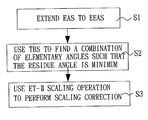•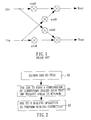•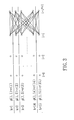•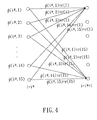•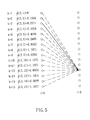•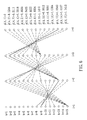•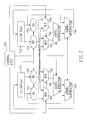•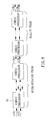•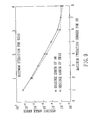•## Classifications

• GPHYSICS
• G06COMPUTING; CALCULATING; COUNTING
• G06FELECTRIC DIGITAL DATA PROCESSING
• G06F7/00Methods or arrangements for processing data by operating upon the order or content of the data handled
• G06F7/38Methods or arrangements for performing computations using exclusively denominational number representation, e.g. using binary, ternary, decimal representation
• G06F7/48Methods or arrangements for performing computations using exclusively denominational number representation, e.g. using binary, ternary, decimal representation using non-contact-making devices, e.g. tube, solid state device; using unspecified devices
• G06F7/544Methods or arrangements for performing computations using exclusively denominational number representation, e.g. using binary, ternary, decimal representation using non-contact-making devices, e.g. tube, solid state device; using unspecified devices for evaluating functions by calculation
• G06F7/5446Methods or arrangements for performing computations using exclusively denominational number representation, e.g. using binary, ternary, decimal representation using non-contact-making devices, e.g. tube, solid state device; using unspecified devices for evaluating functions by calculation using crossaddition algorithms, e.g. CORDIC

## Abstract

A CORDIC method and a CORDIC architecture applied in vector rotation are disclosed. An elementary angles set is extended by representing the elementary angles as the arctangent of the sum of two single signed-power-of-two terms to an extended elementary angles set. A combination of elementary angles is found from the extended elementary angles set such that the residue angle error can be minimized. A quantized scaling factor is used to scale the combination of elementary angles after being micro-rotated.

## Description

BACKGROUND OF THE INVENTION
• 1. Field of the Invention 
• The present invention relates to a COordinate Rotational DIgital Computer (CORDIC) method and a CORDIC architecture applied in vector rotation, and more particularly, to a CORDIC method based on Extended Elementary-Angle Sets (EEAS) and Trellis-based Searching (TBS) and its architecture. 
• 2.Description of Related Art 
• Currently, vector rotation is the kernel of various digital signal-processing applications, including discrete orthogonal transformations, lattice-based digital filtering, matrix computation, complex-valued number manipulation, etc. In X-Y coordinates one vector rotation operation can be expressed as:  $[ x out y out ] = [ cos   θ - sin   θ sin   θ cos   θ ] · [ x in y in ]$
• FIG. 1 shows hardware architecture used to implement the vector rotation operation, which requires four adders and two multipliers. That is, the execution of one vector rotation operation will require executing four addition operations and two multiplication operations. The complexity of such an execution is too high. Reducing the wordlength can decrease the complexity. However, the SQNR will be increased accordingly. 
• The CORDIC algorithm is a well-known iterative method for the computation of vector rotation, which requires only one shift operation and one addition operation to perform a vector rotation operation. The CORDIC algorithm decomposes the rotation angle θ into a combination of pre-defined elementary angles as follows:  $θ = ∑ i = 0 N - 1  μ  ( i )  a  ( i ) + ɛ , ( 1 )$
• where N is the number of elementary angles, μ={1, −1} is the rotation 5 sequence which determines the direction of the i-th elementary angle of a(i)=tan −1(2−i), and ε denotes the residue angle.
• Based on equation (1), the recurrence equations of the CORDIC algorithm can be written as  ${ x  ( i + 1 ) = x  ( i ) - μ  ( i )  y  ( i )  2 - i y  ( i + 1 ) = y  ( i ) + μ  ( i )  x  ( i )  2 - i , ( 2 )$
• for i=0, 1, . . . , N−1. In practical fixed-point implementation, for data wordlength of W bits, no more than W iterations of the recurrence relation in equation (2) need be performed, i.e., N≦W. Also, the final values, x(N) and y(N), need to be scaled by an accumulated scaling factor expressed as follows:  $P = ( ∏ i = 0 N - 1  1 + 2 - 2  i ) - 1 . ( 3 )$
• In the above CORDIC algorithm, each elementary angle needs to be performed sequentially so as to complete the micro-rotation phase. However, in the applications where the rotation angles are known in advance, it would be advantageous to relax the sequential constraint on the micro-rotation phase. The angle recoding (AR) technique is done by extending the set of μ(i) from {1, −1} to {1, −1, 0}. By substituting μ(i)=0 into equation (2), one can skip the micro-rotation of the elementary angle a(i )=tan −1(2−i). Nevertheless, the AR technique imposes no restriction on the iteration number. Rotation angles of different values may need unequal numbers of iterations, which may lead to bus/timing alignment problems in VLSI circuits. Therefore, it is desired for the above CORDIC algorithm to be improved to mitigate and/or obviate the aforementioned problems.
• SUMMARY OF THE INVENTION
• The object of the present invention is to provide a CORDIC method and a CORDIC architecture applied in vector rotation, which can effectively improve SQNR performance, reduce the number of iterations and decrease the hardware complexity. 
• In accordance with one aspect of the present invention, a CORDIC method applied in vector rotation is provided, which first extends an elementary angles set: 
• S 1={tan−1(a′*2−s′ 1 ):a′∈{−1,0,1},s′∈{0,1, . . . ,N−1}},
• by representing the elementary angles as the arctangent of the sum of two single signed-power-of-two (SPT) terms (a′*2 −s′) to an extended elementary angles set:
• s 2={tan −1(a′ 0*2−s′ 0 +a′ 1*2−s′ 1 ):a′ 0 ,a′ 1∈{−1,0,1,},s′ 0 ,s′ 1∈{0,1, . . . ,N−1}},
• where N is the number of elementary angles. Next, a combination of elementary angles is found from the extended elementary angles set such that the residue angle error:  $ɛ m = | θ - ∑ j = 0 R m - 1  tan - 1 [ a 0  ( j ) * 2 - S 0  ( j ) + a 1  ( j ) * 2 - S 1  ( j ) | ,$
• can be minimized, where θ is a target angle; R m is the maximum iteration number; j denotes the iteration index; s0(j), s1(j)∈{0, 1, . . . , N−1} are the rotational sequences; a0(j), a1(j) control the direction of j-th micro-rotation of 2−S 0 (j), 2−S 1 (j). Then, a quantized scaling factor is used to scale the combination of elementary angles determined in step (B) after being micro-rotated.
• In accordance with another aspect of the present invention, a CORDIC processor applied in vector rotation is provided for performing micro-rotational phase operations:  ${ x  ( j + 1 ) = x  ( j ) - [ a 0  ( j ) * 2 - S 0  ( j ) + a 1  ( j ) * 2 - S 1  ( j ) ]  y  ( j ) y  ( j + 1 ) = y  ( j ) + [ a 0  ( j ) * 2 - S 0  ( j ) + a 1  ( j ) * 2 - S 1  ( j ) ]  x  ( j ) , and$
• scaling phase operations:  ${ x ~  ( m + 1 ) = x ~  ( m ) + [ k 0  ( m ) · 2 - q 0  ( m ) + k 1  ( m ) · 2 - q 1  ( m ) ] y ~  ( m + 1 ) = y ~  ( m ) + [ k 0  ( m ) · 2 - q 0  ( m ) + k 1  ( m ) · 2 - q 1  ( m ) ] .$
• In the CORDIC processor, a first register is provided for temporarily storing x(i) or {tilde over (x)}(m). A second register is provided for temporarily storing y(i) or {tilde over (y)}(m) . First and second barrel shifters have input terminals connected to output terminals of the first register. Third and fourth barrel shifters have input terminals connected to output terminals of the second register. A first multiplexer has a first input terminal connected to an output terminal of the first barrel shifter, and a second input terminal connected to an output terminal of the third barrel shifter. A second multiplexer has a first input terminal connected to an output terminal of the second barrel shifter, and a second input terminal connected to an output terminal of the fourth barrel shifter. A third multiplexer has a first input terminal connected to the output terminal of the first barrel shifter, and a second input terminal connected to an output terminal of the third barrel shifter. A fourth multiplexer has a first input terminal connected to the output terminal of the second barrel shifter, and a second input terminal connected to the output terminal of the fourth barrel shifter. A first adder/subtractor has two input terminals connected to output terminals of the first and second multiplexers, respectively. A second adder/subtractor has two input terminals connected to output terminals of the first adder/subtractor and first register, respectively, and an output terminal connected to an input terminal of the first register. A third adder/subtractor has two input terminals connected to output terminals of the third and fourth multiplexers, respectively. A fourth adder/subtractor has two input terminals connected to output terminals of the third adder/subtractor and second register, respectively, and an output terminal connected to an input terminal of the second register. A control unit controls the first to fourth barrel shifters, the first to fourth multiplexers, and the first to fourth adders/subtractors to perform the CORDIC operation iteratively. 
• In accordance with still another object of the present invention, a CORDIC architecture applied in vector rotation is provided having (R m+Rs) CORDIC processors connected in cascade form, in which the Rm leading processors are used in the micro-rotation phase and the following Rs processors are used in the scaling phase.
• Other objects, advantages, and novel features of the invention will become more apparent from the following detailed description when taken in conjunction with the accompanying drawings.
• BRIEF DESCRIPTION OF THE DRAWINGS
• FIG. 1 shows a conventional hardware architecture for implementing vector rotation; 
• FIG. 2 is the flowchart of the CORDIC method in accordance with the present invention; 
• FIG. 3 schematically illustrates the initialization and transfer path of the TBS algorithm; 
• FIG. 4 schematically illustrates an accumulation step example in the TBS algorithm; 
• FIG. 5 schematically illustrates a selection step example in the TBS algorithm; 
• FIG. 6 schematically illustrates a trace back step example in the TBS algorithm; 
• FIG. 7 shows a CORDIC architecture hardware implementation in accordance with the present invention; 
• FIG. 8 shows another CORDIC architecture hardware implementation in accordance with the present invention; 
• FIG. 9 shows a performance comparison between EEAS and EES; and 
• FIG. 10 shows a performance comparison between the TBS method and the Greedy method.
• DETAILED DESCRIPTION OF THE PREFERRED EMBODIMENT
• FIG. 2 shows the flowchart of the CORDIC method applied in vector rotation in accordance with the present invention. As shown the present method includes the following three stages: (S1) extending the elementary angle set (EAS) to an extended elementary angle set (EEAS) for executing the CORDIC algorithm; (S2) using a Trellis-based Searching (TBS) method to find a combination of elementary angles from the elementary angles of the EEAS such that the residue angle is minimum; and (S3) using an extended type-II (ET-II) scaling operation to perform the scaling correction. 
• To depict the EEAS in the first stage, a parameter R m is employed to be the maximum iteration number in the AR technique. Therefore, the AR problem can be summarized as:
• Given a target angle θ and the maximum iteration number R m, find the rotation sequence μ(i)∈{1,−1,0} for 0≦i≦N−1, such that the residue angle error: $ɛ m ≡ | θ - ∑ i = 0 N - 1  μ  ( i )  a  ( i ) | = | θ - ∑ i = 0 N - 1  μ  ( i )   tan - 1  ( 2 - i ) | , ( 4 )$
• is minimized subject to the constraint:  $∑ i = 0 N - 1  | μ  ( i ) | ≤ R m . ( 5 )$
• To facilitate the derivation of the present EEAS scheme, the AR problem described above is rewritten in an alternative form. The reformulation is done by removing the redundant iterations of μ(i)=0 in equation (4), changing the variables and index, and using the equality ±tan −1(A)=tan−1(±A). Then, the equations (4) and (5) can be recast in a single compact form as: $ɛ m = | θ - ∑ j = 0 R m - 1  tan - 1 ( a  ( j ) * 2 - s  ( j ) ) | = | θ - ∑ j = 0 R m - 1  θ ′  ( j ) | , ( 6 )$
• where j(0≦j≦R m−1) denotes the iteration index; s(j)∈{0, 1, . . . , N−1} is the rotational sequence that determines the micro-rotation angle in the j-th iteration; a(j)∈{−1, 0, 1} is the directional sequence that controls the direction of the j-th micro-rotation of 2−s(j):θ′(j) is the j-th micro-rotation angle and defined as θ′(j)≡tan−1(a(j)*2−s(j)).
• Therefore, equation (6) shows that the AR problem is finding the combination of elements from a set, which consists of all possible values of θ′(j), so that ε m can be minimized. Such a set is called the elementary angles set (EAS) S1, and is defined as
• S 1={tan−1(a′*2−s′):a′∈{−1,0,1},s′∈{0,1, . . . ,N−1}}.   (7)
• By observing equation (7), it is seen that the EAS S1 is comprised of an arctangent of single signed-power-of-two (SPT) term, i.e., tan −1(a′*2−s′). By representing the elementary angles as the arctangent of the sum of two SPT terms, the EAS S1 can be extended to an extended elementary angles set (EEAS) S2 as follows:
• s 2={tan−1(a′ 0*2−s′ 0 +a′ 1*2−s′ 1 ):a′ 0 ,a′ 1∈{−1,0,1{,s′ 0 ,s′ 1∈{0,1, . . . ,N−1}}.   (8)
• Based on the EEAS S 2 developed in equation (8), the recurrence equations of the CORDIC algorithm can be modified as: ${ x  ( j + 1 ) = x  ( j ) - [ a 0  ( j ) * 2 - S 0  ( j ) + a 1  ( J ) * 2 - S 1  ( j ) ]  y  ( j ) y  ( j + 1 ) = y  ( j ) + [ a 0  ( j ) * 2 - S 0  ( j ) + a 1  ( j ) * 2 - S 1  ( j ) ]  x  ( j ) , ( 9 )$
• for 0≦j≦R−1, where a 0(j), a1(j), s0(j) and s1(j) denote the parameters to control the j-th micro-rotation of the elementary angle of tan−1(a0(j)*2−S 0 (j)+a1(j)*2−S 1 (j)).
• With the above EEAS S 2 and given θ and Rm, it is desired to find the parameters of a0(j), a1(j), s0(j) and s1(j) (i.e., the combination of elementary angles from EEAS S2), such that the residue angle error: $ɛ m = | θ - ∑ j = 0 R m - 1  tan - 1 [ a 0  ( j ) * 2 - S 0  ( j ) + a 1  ( j ) * 2 - S 1  ( j ) | , ( 10 )$
• can be minimized. 
• In the second stage of processing, the TBS algorithm is employed to find the combination of elementary angles, wherein Z(S 2) denotes the number of the elementary angles in the extended set S2, and each distinct elementary angle in the set is expressed as r(k), for 1≦k≦Z(S2), i.e., S2={r(1), r(2), . . . , r(Z(S2))}. Moreover, in the TBS algorithm, there are Z(S2) states in each step. For the k-th state (1≦k≦Z(S2)) of the i-th search step, a cumulative angle Φ(i,k) is used to denote the best approximation of angle θ in the k-th state up to the i-th step. The TBS algorithm can be described as follows:
• (1) Initialization Step: 
• The TBS algorithm is started by setting all Φ(i,k) as the corresponding elementary angles, that is: 
• Φ(1,k)=r(k) for all k.   (11)
• Taking Z(S 2)=15 as an example, the initialization and transfer paths of the TBS algorithm are illustrated in FIG. 3.
• (2) Accumulation Step 
• A path in the trellis, which leaves the k-th state at the i-th step and enters the k-th state at the (i+1)-th step, corresponds to an operation of Φ(i,k)+r(k′). For all paths, the appended angle of Φ(i,k)+r(k′) becomes the candidate for Φ(i+1,k′). As shown in FIG. 4, from a given state at step i=i*, the paths can diverge to all the states at the next search step (i*+1). Namely, there are Z(S 2) paths, carrying the corresponding appended angles of Φ(i,k)+r(k′) for all k, entering the k′-th state at the (i*+1)-th step. Then, those appended angles form the candidate set for the cumulative angle of Φ(i*+1,k′).
• (3) Comparison and Selection Step 
• The TBS algorithm involves calculating and minimizing the difference between the target angle θ and Φ(i,k) for all k at each search step i. To be specific, Φ(i+1,k) is determined by: 
• Φ(i+1,k)=min{|Φ(i,k*)+r(k)−θ|:1≦k*≦Z(S 2){.   (12)
• Then, the selected path is denoted as the surviving path. After calculating all the cumulative angles Φ(i,k) for all k, their corresponding surviving paths can be obtained. Continuing in this manner and moving to the (i+1)-th step until reaching the maximum iteration number (i=Rm), Φ(R m,k) can be obtained for 1≦k≦Z(S2). Consider the example, in which i=2 and k=12. The process of equation (12) is illustrated in FIG. 5. From those 15 paths entering the 12th state at the 3rd search step, the path, of which the appended angle Φ(2, j)+r(12) is closest to θ=π/3, is selected as the surviving path. In this case, the third path (k*=3), which is marked by the solid line, is selected. Then, the resultant angle is assigned to Φ(3,12) for the subsequent search process.
• (4) Determination of the Global Result and Trace Back Step 
• After calculating the cumulative angles for all states at the last search step, i.e., Φ(R m,k) for 1≦k≦Z(S2), the next procedure for the TBS algorithm is to determine the global result, θTBS. Similar to the determination of the surviving path, the θTBS is decided as follows:
• θTBS =min{|Φ(R m ,K′)−θ|:1≦k′≦Z(S 2){.   (13)
• Next, all the micro-rotations can be determined by tracing back from the state, whose corresponding Φ(R m,k) is the best approximation of θ, along with its surviving backward path.
• The procedure for trace back is illustrated in FIG. 6. All the surviving paths for each state at each step (except i=1) are represented by the dashed line. First, Φ(4,13) is selected as the global result. Then, it is traced along the surviving path that connects the 13th state at the final backward step. Next, it finds the state from which the surviving path leaves in the previous step. By doing the process repeatedly, the global surviving path of the TBS algorithm can be determined, as marked by the solid line in. By traveling along the surviving global path, the algorithm is able to find the visited states and read all the micro-rotation angles that form the global result θ TBS. In this case, Φ(4,13)=r(9)+r(13)+r(2)+r(13), which is the best approximation of angle θ generated by the present TBS algorithm.
• When considering that each micro rotation will scale up the norm of a vector, in the known type-II scaling operation, the accumulated scaling factor P is quantized as:  $p ^ = ∏ m = 0 R s - 1   ( 1 + k  ( m ) · 2 q  ( m ) ) , ( 14 )$
• where {circumflex over (P)} denotes the quantized value of P,  k(m)∈{1,−} and q(m)∈{0,1, . . . ,W−1, is the counterpart of Rm in the micro-rotation phase, determining the number of iterations in the scaling phase. With this type-II scaling operation, and by increasing the number of possible values that can be represented by (1+k(m)·2 −q(m) ), we obtain a similar derivation of the EEAS scheme that employs one extra SPT term in equation (14): $P ^ = ∏ m = 0 R s - 1   ( 1 + k 0  ( m ) · 2 - q 0  ( m ) + k 1  ( m ) · 2 - q 1  ( m ) ) , ( 15 )$
• where
• k 0 (m)|{1,−1,0},k 1 (m)∈{1,−1,0},q 0 (m)∈{0,1, . . . ,W−1},
• and 
• q 1 (m)∈{0,1, . . . ,W−1}.
• By doing so, it is expected to obtain more accurate approximations of P. The scaling operation can be accomplished within R s, iterations by using the recurrence equations: $ { x ~  ( m + 1 ) = x ~  ( m ) + [ k 0  ( m ) · 2 - q 0  ( m ) + k 1  ( m ) · 2 - q 1  ( m ) ] y ~  ( m + 1 ) = y ~  ( m ) + [ k 0  ( m ) · 2 - q 0  ( m ) + k i  ( m ) · 2 - q 1  ( m ) ] , ( 16 )$
• wherein the initial settings for the scaling phase are set as {tilde over (x)}(0)=x(R m) and {tilde over (y)}(0)=y(Rm).
• FIG. 7 shows a hardware implementation of the CORDIC architecture applied in vector rotation according to the present invention, which is an iterative CORDIC processor. It consists of a control unit  701, first and second registers 711 and 712, first to fourth barrel shifters 721˜724, first to fourth multiplexers 731˜734, first to fourth adders/subtracters 741˜744.
• The first register  711 is provided for temporarily storing x(i) or {tilde over (x)}(m), and its output terminal is connected to the first and second barrel registers 721 and 722, and its input terminal is connected to the second adder/subtracter 742. The second register 712 is provided for temporarily storing y(i) or {tilde over (y)}(m), and its output terminal is connected to the third and fourth barrel registers 723 and 724, and its input terminal is connected to the fourth adder/subtractor 744.
• The output terminal of the first barrel register  721 is connected to the first input terminal of the first multiplexer 731 and the third multiplexer 733. The output terminal of the second barrel register 722 is connected to the first input terminal of the second multiplexer 732 and the fourth multiplexer 734. The output terminal of the third barrel register 723 is connected to the second input terminal of the first multiplexer 731 and the third multiplexer 733. The output terminal of the fourth barrel register 724 is connected to the second input terminal of the second multiplexer 732 and the fourth multiplexer 734.
• The output terminals of the first and second multiplexers  731 and 732 are connected to the input terminals of the first adder/subtractor 741. The output terminal of the first adder/subtractor 741 is connected to the input terminal of the second adder/subtractor 742. The output terminals of the third and fourth multiplexers 733 and 734 are connected to the input terminals of the third adder/subtractor 743. The output terminal of the third adder/subtractor 743 is connected to the input terminal of the fourth adder/subtractor 744. The output terminals of the second and fourth adders/subtractors 742 and 744 are connected to the input terminals of the first and second register 711 and 712, respectively.
• The control unit  701 is provided to control the first to fourth barrel shifters 721˜724, the first to fourth multiplexers 731˜734, and the first to fourth adders/subtractors 741˜744, so as to implement the CORDIC algorithm. Two separate phases are performed to complete a single CORDIC rotation, i.e., the micro-rotational phase and the scaling phase. In the micro-rotational phase, under the control of the control unit 701, the first and second barrel shifters 721 and 722 are shifted by s1(i) and s0(i) bits, respectively. The third and fourth barrel shifters 723 and 724 are shifted by s0(i) and s1(i) bits, respectively. The second input terminals of the first and second multiplexers 731 and 732 are switched to connect to their output terminals thereof, respectively. The first input terminals of the third and fourth multiplexers 733 and 734 are switched to connect to their output terminals thereof, respectively. The control unit 701 uses a0(i) and a1(i) to control first to fourth adders/subtracters 741˜744, so as to perform addition and subtraction.
• In the scaling phase, under the control of the control unit  701, the first and second barrel shifters 721 and 722 are shifted by q1(m) and q0(m) bits, respectively. The third and fourth barrel shifters 723 and 724 are shifted by q0(m) and q1(m) bits, respectively. The first input terminals of the first and second multiplexers 731 and 732 are switched to connect to their output terminals thereof, respectively. The second input terminals of the third and fourth multiplexers 733 and 734 are switched to connect to their output terminals thereof, respectively. The control unit 701 uses k0(m) and k1(m) to control the first to fourth adders/subtracters 741˜744, so as to perform addition and subtraction.
• By unfolding the iterative implementation of the above CORDIC processor, a parallel structure can be obtained as depicted in FIG. 8. The structure is composed of (R m+Rs) CORDIC processors 81 connected in cascade form, in which the Rm leading processors 81 perform the micro-rotations and the following Rs processors 81 execute the scaling operations. Each CORDIC processor 81 performs one iteration as specified in FIG. 7.
• In view of the foregoing, it is known that the present invention performs the CORDIC algorithm by extending EAS to EEAS. As shown in FIG. 9 that gives the comparison of the maximum iteration number and residue angle error between EEAS and EAS, it is known that EEAS can have a smaller residue angle error with less iteration number. FIG. 10 shows an experimental result in which the TBS method requires a smaller iteration number than the conventional Greedy method, and the combination of EEAS and TBS methods can further reduce the iteration number. 
• Although the present invention has been explained in relation to its preferred embodiment, it is to be understood that many other possible modifications and variations can be made without departing from the spirit and scope of the invention as hereinafter claimed. 

## Claims (12)

What is claimed is:
1. A CORDIC method applied in vector rotation comprising the steps of:
(A) extending an elementary angles set:
S 1={tan−1(a′*2−s′):a′∈{−1,0,1{,s′∈{0,1, . . . ,N−1}},
by representing the elementary angles as the arctangent of the sum of two single signed-power-of-two (SPT) terms (a′*2−s′) to an extended elementary angles set:
s 2={tan−1(a′ 0*2−s′ 0 +a′ 1*2−s′ 1 ):a′ 0 ,a′ 1∈{−1,0,1},s′ 0 ,s′ 1∈{0,1, . . . ,N−1}},
where N is the number of elementary angles;
(B) finding a combination of elementary angles from the extended elementary angles set such that the residue angle error:
$ɛ m = | θ - ∑ j = 0 R m - 1  tan - 1 [ a 0  ( j ) * 2 - S 0  ( j )  a 1  ( j ) * 2 - S 1  ( j ) | ,$
can be minimized, where θ is a target angle; Rm is the maximum iteration number; j denotes the iteration index; s0(j),s1(j),∈{0,1, . . . , N−1} are the rotational sequences; a0(j), a1(j), control direction of j-th micro-rotation of 2−S 0 (j), 2−S 1 (j); and
(C) using a quantized scaling factor to scale the combination of elementary angles determined in step (B) after being micro-rotated.
2. The method as claimed in claim 1, wherein, based on the extended elementary angles set of step (A), the CORDIC method is performed by the recurrence equations:
$x  ( j + 1 ) = x  ( j ) - [ a 0  ( j ) * 2 - s 0  ( j ) + a 1  ( j ) * 2 - s 1  ( j ) ]  y  ( j ) y  ( j + 1 ) = y  ( j ) + [ a 0  ( j ) * 2 - s 0  ( j ) + a 1  ( j ) * 2 - s 1  ( j ) ]  x  ( j ) ,$
for 0≦j≦Rm−1, where a0(j), a1(j), s0(j) and s1(j) denote parameters to control the j-th micro-rotation of tan−1(a0(j)*2−S 0 (j)+a1(j)*2−S 1 (j))′s elementary angle.
3. The method as claimed in claim 1, wherein, in step (B), a Trellis-based Searching (TBS) method is employed to find the combination of elementary angles, and the TBS method uses a cumulative angle Φ(i,k) to denote the best approximation of angle θ in a k-th state up to an i-th step; the TBS method comprises the steps of:
(B1) setting all Φ(i,k) as the corresponding elementary angles r(k);
(B2) for a path which leaves the k-th state at the i-th step and enters the k-th state at the (i+1)-th step, corresponding to an operation of Φ(i,k)+r(k′), and for all paths, taking Φ(i,k)+r(k′) as the candidate for Φ(i+1,k′)
(B3) comparing all candidates for Φ(i+1,k) at each search step, so as to select a candidate closest to the target angle θ as Φ(i+1,k) and record the corresponding path as a surviving path; and
(B4) after calculating the cumulative angles for all states at the last search step, selecting a cumulative angle closest to the target angle θ for use as a global result θTBS, and tracing along its surviving path backward to determine all micro-rotations.
4. The method as claimed in claim 3, wherein, in step (B3), Φ(i+1,k) is determined by:
Φ(i+1,k)=min {|Φ(i,k*)+r(k)−θ|:1≦k*≦Z(S 2)},
where Z(S2) denotes the number of elementary angles in an extended set S2.
5. The method as claimed in claim 4, wherein, in step (B4), θTBS is decided by:
θTBS =min {|Φ(R m ,k′)−θ|:1≦k′≦Z(S 2)}.
6. The method as claimed in claim 1, wherein, in step (C), the quantized scaling factor is:
$P ^ = ∏ m = 0 R s - 1   ( 1 + k 0  ( m ) · 2 - q 0  ( m ) + k 1  ( m ) · 2 - q 1  ( m ) ) ,$
where k0(m)∈{1,−1,0}, k1(m)∈{1,−1,0}, q0(m)∈{0,1, . . . ,W−1}, q1(m)∈{0,1, . . . ,W−1}, and Rs is a counterpart of Rm in the micro-rotation phase.
7. The method as claimed in claim 6, wherein step (C) accomplishes scaling operations within R, iterations by using recurrence equations:
$x ~  ( m + 1 ) = x ~  ( m ) + [ k 0  ( m ) · 2 - q 0  ( m ) + k 1  ( m ) · 2 - q 1  ( m ) ] y ~  ( m + 1 ) = y ~  ( m ) + [ k 0  ( m ) · 2 - q 0  ( m ) · + k 1  ( m ) · 2 - q 0  ( m ) ] .$
8. The method as claimed in claim 6, wherein the scaling operation is initialized as {tilde over (x)}(0)=x(R m ) and {tilde over (y)}(0)=y(R m ).
9. A CORDIC processor applied in vector rotation for performing micro-rotational phase operations:
$x  ( j + 1 ) = x  ( j ) - [ a 0  ( j ) * 2 s 0  ( j ) + a 1  ( j ) * 2 - s 1  ( j ) ]  y  ( j ) y  ( j + 1 ) = y  ( j ) + [ a 0  ( j ) * 2 - s 0  ( j ) + a 1  ( j ) * 2 s 1  ( j ) ]  x  ( j ) , and$
scaling phase operations:
${ x ~  ( m + 1 ) = x ~  ( m ) + [ k 0  ( m ) · 2 - q 0  ( m ) + k 1  ( m ) · 2 - q 1  ( m ) ] y ~  ( m + 1 ) = y ~  ( m ) + [ k 0  ( m ) · 2 - q 0  ( m ) + k 1  ( m ) · 2 - q 1  ( m ) ] ,$
the processor comprising:
a first register for temporarily storing x(i) or {tilde over (x)}(m);
a second register for temporarily storing y(i) or {tilde over (y)}(m);
first and second barrel shifters having input terminals connected to output terminals of the first register;
third and fourth barrel shifters having input terminals connected to output terminals of the second register;
a first multiplexer having a first input terminal connected to an output terminal of the first barrel shifter, and a second input terminal connected to an output terminal of the third barrel shifter;
a second multiplexer having a first input terminal connected to an output terminal of the second barrel shifter, and a second input terminal connected to an output terminal of the fourth barrel shifter;
a third multiplexer having a first input terminal connected to the output terminal of the first barrel shifter, and a second input terminal connected to an output terminal of the third barrel shifter;
a fourth multiplexer having a first input terminal connected to the output terminal of the second barrel shifter, and a second input terminal connected to the output terminal of the fourth barrel shifter;
a first adder/subtractor having two input terminals connected to output terminals of the first and second multiplexers, respectively;
a second adder/subtractor having two input terminals connected to output terminals of the first adder/subtractor and first register, respectively, and an output terminal connected to an input terminal of the first register;
a third adder/subtractor having two input terminals connected to output terminals of the third and fourth multiplexers, respectively;
a fourth adder/subtractor having two input terminals connected to output terminals of the third adder/subtractor and second register, respectively, and an output terminal connected to an input terminal of the second register; and
a control unit for controlling the first to fourth barrel shifters, the first to fourth multiplexers, and the first to fourth adders/subtractors to perform CORDIC operations iteratively.
10. The processor as claimed in claim 9, wherein, in the micro-rotational phase, the first and second barrel shifters are shifted by s1(i) and s0(i) bits, respectively; the third and fourth barrel shifters are shifted by s0(i) and s1(i) bits, respectively; the second input terminals of the first and second multiplexers are switched to connect to their output terminals, respectively; the first input terminals of the third and fourth multiplexers are switched to connect to their output terminals, respectively; the first to fourth adders/subtracters perform addition and subtraction under the control of a0(i) and a1(i).
11. The processor as claimed in claim 9, wherein, in the scaling phase, the first and second barrel shifters are shifted by q1(m) and q0(m) bits, respectively; the third and fourth barrel shifters are shifted by q0(m) and q1(m) bits, respectively; the first input terminals of the first and second multiplexers are switched to connect to their output terminals, respectively; the second input terminals of the third and fourth multiplexers are switched to connect to their output terminals, respectively; the first to fourth adders/subtracters perform addition and subtraction under the control of k0(m) and k1(m).
12. A CORDIC architecture applied in vector rotation comprising (Rm+Rs) CORDIC processors as claimed in claim 9 connected in cascade form, in which the Rm leading processors are used in the micro-rotational phase and the following Rs processors are used in the scaling phase.
US10/138,652 2001-10-31 2002-05-06 Cordic method and architecture applied in vector rotation Active 2023-12-24 US7047269B2 (en)

## Priority Applications (2)

Application Number Priority Date Filing Date Title
TW90127085 2001-10-31
TW90127085A TW583579B (en) 2001-10-31 2001-10-31 Computation method and structure of coordinate rotational digital computer applied in vector rotator

## Publications (2)

Publication Number Publication Date
US20030097388A1 true US20030097388A1 (en) 2003-05-22
US7047269B2 US7047269B2 (en) 2006-05-16

# Family

## Family Applications (1)

Application Number Title Priority Date Filing Date
US10/138,652 Active 2023-12-24 US7047269B2 (en) 2001-10-31 2002-05-06 Cordic method and architecture applied in vector rotation

## Country Status (2)

Country Link
US (1) US7047269B2 (en)
TW (1) TW583579B (en)

## Cited By (7)

* Cited by examiner, † Cited by third party
Publication number Priority date Publication date Assignee Title
US20050198089A1 (en) * 2004-03-08 2005-09-08 Industrial Technology Research Institute Mixed-scaling-rotation CORDIC method with scaling-free rotational operations for vector rotation
US20060059215A1 (en) * 2001-12-20 2006-03-16 Koushik Maharatna Cordic unit
US20070155311A1 (en) * 2000-09-13 2007-07-05 Stratosaudio, Inc. Broadcast response system
US20080140239A1 (en) * 2000-03-08 2008-06-12 Music Choice Personalized Audio System and Method
CN102323878A (en) * 2011-05-31 2012-01-18 电子科技大学 Circuit device and method for norm correction of CORDIC (Coordinated Rotation Digital Computer) algorithm
CN103914625A (en) * 2014-04-10 2014-07-09 电子科技大学 Wireless signal direction-finding method based on CORDIC (coordinated rotation digital computer) algorithm
US9591051B2 (en) 2000-03-08 2017-03-07 Music Choice Systems and methods for providing customized media channels

## Families Citing this family (5)

* Cited by examiner, † Cited by third party
Publication number Priority date Publication date Assignee Title
US20050216540A1 (en) * 2004-03-25 2005-09-29 Wen Kuei-Ann Novel CORDIC circuit
WO2009034517A2 (en) * 2007-09-10 2009-03-19 St-Nxp Wireless (Holding) Ag Barrel shifter unit and method of barrel shifting
JP5310720B2 (en) * 2007-09-26 2013-10-09 日本電気株式会社 fft and ifft apparatus and method based on Cordic
TWI376633B (en) * 2008-12-19 2012-11-11 Ind Tech Res Inst Method of cordic computing vector angle and electronic apparatus using the same
RU2683182C1 (en) * 2017-12-21 2019-03-26 Акционерное общество "Ангстрем" (АО "Ангстрем") Asynchronous vector turn device

## Citations (2)

* Cited by examiner, † Cited by third party
Publication number Priority date Publication date Assignee Title
US20030050949A1 (en) * 2000-09-12 2003-03-13 Van Wechel Robert J. Simultaneous computation of multiple complex number multiplication products
US20030206600A1 (en) * 1999-04-23 2003-11-06 Nokia Networks Oy QAM Modulator

## Patent Citations (3)

* Cited by examiner, † Cited by third party
Publication number Priority date Publication date Assignee Title
US20030206600A1 (en) * 1999-04-23 2003-11-06 Nokia Networks Oy QAM Modulator
US20030050949A1 (en) * 2000-09-12 2003-03-13 Van Wechel Robert J. Simultaneous computation of multiple complex number multiplication products
US20040039761A1 (en) * 2000-09-12 2004-02-26 Wechel Robert J. Van Systems and methods for extracting coherent correlation data

## Cited By (9)

* Cited by examiner, † Cited by third party
Publication number Priority date Publication date Assignee Title
US9591051B2 (en) 2000-03-08 2017-03-07 Music Choice Systems and methods for providing customized media channels
US20080140239A1 (en) * 2000-03-08 2008-06-12 Music Choice Personalized Audio System and Method
US8051146B2 (en) 2000-03-08 2011-11-01 Music Choice Personalized audio system and method
US20070155311A1 (en) * 2000-09-13 2007-07-05 Stratosaudio, Inc. Broadcast response system
US20060059215A1 (en) * 2001-12-20 2006-03-16 Koushik Maharatna Cordic unit
US7606852B2 (en) * 2001-12-20 2009-10-20 IHP-GmbH-Innovations for High Performance Microelectronics/Institut fur Innovative Mikroelectronik CORDIC unit
US20050198089A1 (en) * 2004-03-08 2005-09-08 Industrial Technology Research Institute Mixed-scaling-rotation CORDIC method with scaling-free rotational operations for vector rotation
CN102323878A (en) * 2011-05-31 2012-01-18 电子科技大学 Circuit device and method for norm correction of CORDIC (Coordinated Rotation Digital Computer) algorithm
CN103914625A (en) * 2014-04-10 2014-07-09 电子科技大学 Wireless signal direction-finding method based on CORDIC (coordinated rotation digital computer) algorithm

## Also Published As

Publication number Publication date
US7047269B2 (en) 2006-05-16
TW583579B (en) 2004-04-11

## Similar Documents

Publication Publication Date Title
Despain Very fast Fourier transform algorithms hardware for implementation
US7930335B2 (en) Generic implementations of elliptic curve cryptography using partial reduction
US6219688B1 (en) Method, apparatus and system for sum of plural absolute differences
Andraka A survey of CORDIC algorithms for FPGA based computers
US4969118A (en) Floating point unit for calculating A=XY+Z having simultaneous multiply and add
US5995748A (en) Three input arithmetic logic unit with shifter and/or mask generator
Takagi et al. Redundant CORDIC methods with a constant scale factor for sine and cosine computation
US9813224B2 (en) Digital processor having instruction set with complex angle function
US5341321A (en) Floating point arithmetic unit using modified Newton-Raphson technique for division and square root
US5640578A (en) Arithmetic logic unit having plural independent sections and register storing resultant indicator bit from every section
US6058473A (en) Memory store from a register pair conditional upon a selected status bit
US5742538A (en) Long instruction word controlling plural independent processor operations
US6385634B1 (en) Method for performing multiply-add operations on packed data
US5517438A (en) Fast multiply-add instruction sequence in a pipeline floating-point processor
EP1293891B1 (en) Arithmetic processor accomodating different finite field size
US5805913A (en) Arithmetic logic unit with conditional register source selection
US5465224A (en) Three input arithmetic logic unit forming the sum of a first Boolean combination of first, second and third inputs plus a second Boolean combination of first, second and third inputs
US20020013800A1 (en) Method and apparatus for performing single-cycle addition or subtraction and comparison in redundant form arithmetic
US5596763A (en) Three input arithmetic logic unit forming mixed arithmetic and boolean combinations
KR0146334B1 (en) Cordic complex multiplier
Juang et al. Para-CORDIC: Parallel CORDIC rotation algorithm
US6480872B1 (en) Floating-point and integer multiply-add and multiply-accumulate
US4700324A (en) Digital circuit performing an arithmetic operation with an overflow
US5420809A (en) Method of operating a data processing apparatus to compute correlation
US20040148321A1 (en) Method and system for performing calculation operations and a device

## Legal Events

Date Code Title Description
AS Assignment

Owner name: INDUSTRIAL TECHNOLOGY RESEARCH INSTITUTE, TAIWAN

Free format text: ASSIGNMENT OF ASSIGNORS INTEREST;ASSIGNORS:WU, CHENG-SHING;PAN, CHIA-HO;WU, AN-YEU;REEL/FRAME:012869/0665

Effective date: 20020418

FPAY Fee payment

Year of fee payment: 4

FPAY Fee payment

Year of fee payment: 8

MAFP

Free format text: PAYMENT OF MAINTENANCE FEE, 12TH YEAR, LARGE ENTITY (ORIGINAL EVENT CODE: M1553)

Year of fee payment: 12